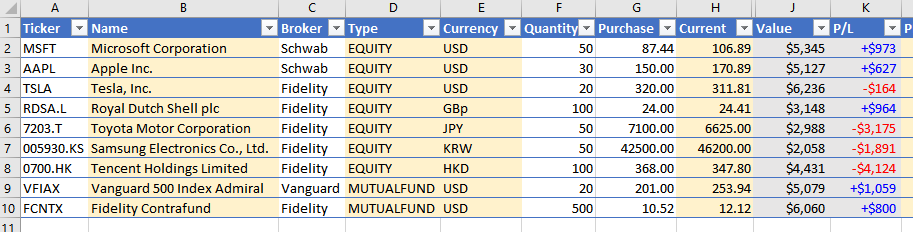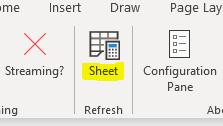## Building an Investment Portfolio in Excel

Excel Price Feed is ideal for helping you build a spreadsheet to model your investment portfolio with live financial data.

##### Introduction

To get started, you can download an example portfolio spreadsheet, with formulas for using live data from Yahoo Finance:

In this example, I am holding a variety of international stocks, denominated in various currencies across several brokers. In addition I hold some US mutual funds:The white cells are the only cells which require any information to be manually entered; the grey cells are autocalculated by Excel and the yellow cells are where live data is provided by the Excel Price Feed Add-in.

As you can see only 4 types of data are required for each investment: ticker, broker, quantity and purchase price.

##### Excel Price Feed Formulas

If you view the formulas in the yellow cells you will see they all are in the format `=EPF.Yahoo` which indicates the formula is an Excel Price Feed formula connected to Yahoo Finance.

Excel Price Feed formulas usually reference a stock/fund ticker which in this example is in the first column.

If you don't know the ticker you can use the search box on the Configuration Pane (accessed from the button on the toolbar)

As the column is in an Excel table we can reference it by the name @ticker. For example the formula behind the Name column is:

`=EPF.Yahoo.Name([@Ticker])`

##### Currency Conversions

The Value column shows the current value of the holding denominated in US Dollars, even though several of the holdings are denominated in other currencies. For example, Samsung Electronics is a South Korean stock denominated in Korean Won.

There is a hidden column, I, which contains the latest USD exchange rate, in this case the KRWUSD exchange rate. The formula in this column is:

``` =EPF.Yahoo.Price([@Currency]&"USD=X") ```

As you can see it references the Currency column to generate the currency pair "KRWUSD" which is then passed into the Excel Price Feed live price formula (as Yahoo instrument code KRWUSD=X) to retrieve the latest exchange rate.

This FX rate value is referenced by the formula in the Value column which results in the value being converted to USD:

`=([@Current]*[@Quantity])*[@FX]`

And the result is a live FX rate and value in USD:##### Data Refresh

Any time you wish to revalue the portfolio based on live prices, simply press the "Refresh Sheet" button on the Excel Price Feed toolbar:##### Summary

We hope this tutorial has provided some useful guidance on using Excel Price Feed to build a "live" investment portfolio in Excel.

Discover the power of Excel Price Feed with 80+ new Excel formulas for live, historic and fundamental data in your spreadsheet. Click the button below to request an Activation Code for your free 10 day trial: